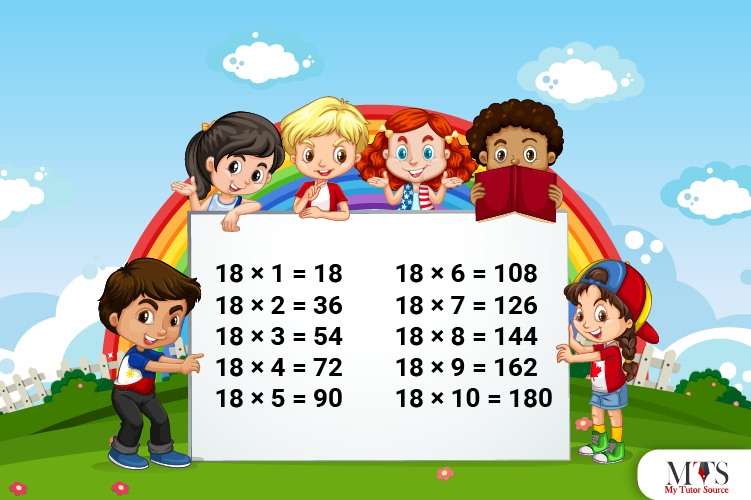# Multiplication Table of 18 – Tips to Memorize 18 Times Table & Example QuestionsMemorizing tables at your fingertips is an essential skill, and every student must master it. Many students find the 18 times table complicated and challenging to learn. It has complex numbers which are hard to calculate and remember. Therefore, in this article, we have provided everything you need to know for memorizing the table of 18. All experienced teachers and competent online and private math tutors use the same quick tips mentioned below.

Let us learn the table of 18

## Table of 18

Here is the table of 18; recite it till you memorize it at your fingertips. Once you learn it effectively – solving lengthy, tricky, and intimidating math problems would be an easy deal for you.

18 x 1 = 18
18 x 2 = 36
18 x 3 = 54
18 x 4 = 72
18 x 5 = 90
18 x 6 = 108
18 x 7 = 126
18 x 8 = 144
18 x 9 = 162
18 x 10 = 180
18 x 11 = 198
18 x 12 = 216
18 x 13 = 234
18 x 14 = 252
18 x 15 = 270
18 x 16 = 288
18 x 17 = 306
18 x 18 = 324
18 x 19 = 342
18 x 20 = 360

## Method to Read Table of 18

Learning and memorizing the table of 18 is easy if you know how to read it. Therefore, we have provided the multiplication table of 18 in words in this section.

Eighteen ones are eighteen (18)
Eighteen twos are thirty-six (36)
Eighteen threes are fifty-four (54)
Eighteen fours are seventy-two (72)
Eighteen fives are ninety (90)
Eighteen sixes are one hundred and eight (108)
Eighteen sevenths are one hundred and twenty-six (126)
Eighteen eighths are one hundred and forty-four (144)
Eighteen nines are one hundred and sixty-two (162)
Eighteen tens are one hundred and eighty (180)
Eighteen elevens are one hundred and ninety-eight (198)
Eighteen twelves are two hundred and sixteen (216)
Eighteen thirteens are two hundred and thirty-four (234)
Eighteen fourteens are two hundred and fifty-two (252)
Eighteen fifteens are two hundred and seventy (270)
Eighteen sixteens are two hundred and eighty-eight (288)
Eighteen seventeens are three hundred and six (306)
Eighteen eighteenths are three hundred and twenty-four (324)
Eighteen nineteens are three hundred and forty-two (342)
Eighteen twenties are three hundred and sixty (360)

## Tips for Memorizing Multiplication Table of 18

Mathematics has rules and patterns, but not always! But do you know that you can obtain and learn 18 times tables in many ways? Read this section to discover a few surprising yet easy tips to memorize 18 times table. Let’s get started!

### Tip # 1: Recall 17 times table

Use the 17 times table and your adding skills to learn the table of 18. Add the natural numbers to its product to generate the product results of the 18 times table. Go through the table given below to find out how:

### Tip # 2: Recall 9 times table

Another trick to memorize the table of 9 is doubling the results of the 9 times table. We advise you to use this tip if you find multiples of 18 times table tricky and hard to remember.

Go through the table mentioned below to learn how to use the 9 times table for quick learning.

### Tip # 3: Recall 8 times table

You can also use an 8 times table and add natural numbers (multipliers) to the tens’ digit of its product to get a table of 18. Go through the table below to better understand this tip for memorizing 18 times table:

### Example Question of Table of 18

Question: If a group of students takes 18 days to complete a project, then how many days will they take to complete 5 such projects?

Solution:

Given that,
Days taken to complete 1 project = 18
Total number of projects = 5
Using table of 18,
⇒ 18 x 5 = 90
Thus, the students will take 90 days to complete 5 projects.

Question: What is the value of eighteen times of eighteen?

Solution:

Given that,
Eighteen times of eighteen
Mathematically it is written as,
⇒ 18 x 18 = 324
Hence, the value of eighteen times eighteen is three hundred and twenty-four.

Question: Find the number after 10 x 18 and before 8 x 18.

Solution:

10 x 18 = 180
Number after 180 = 180 + 1 = 181

And,

8 x 18 = 144
Number before 144 = 144 – 1 = 143

Hence, the number after 10 x 18 is 181, and before 8 x 18 is 143.

Question: By repeated addition, find the cost of 6 chocolates if the price of one chocolate is \$18? (Use table of 18)

Solution:

Given that,
Cost of 1 chocolate = \$18
Total chocolates = 6
By table of 18,
Cost of 6 chocolates = 6 x 18 = 108
Hence, the cost of 6 chocolates is \$ 108.

Question: Find the value of 18 times 9 minus 21?

Solution:
Given that,
18 times 9 minus 21
In mathematical form,
= 18 x 9 – 21
= 342 – 21
= 321
Hence, the value of 18 times 9 minus 21 is 321

### Find Top Tutors in Your AreaWith over 3 years of experience in teaching, Chloe is very deeply connected with the topics that talk about the educational and general aspects of a student's life. Her writing has been very helpful for students to gain a better understanding of their academics and personal well-being. I’m also open to any suggestions that you might have! Please reach out to me at chloedaniel402 [at] gmail.com